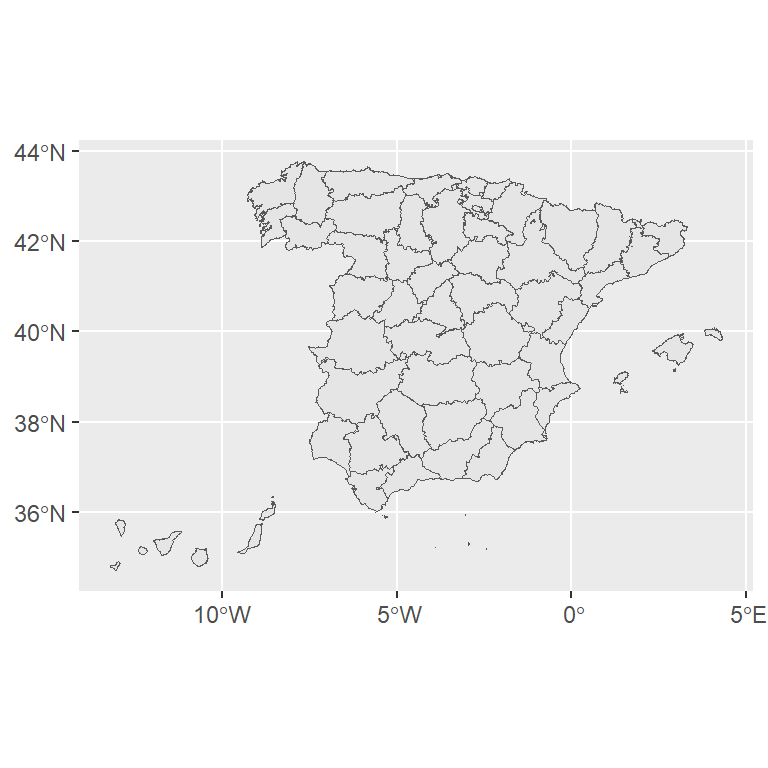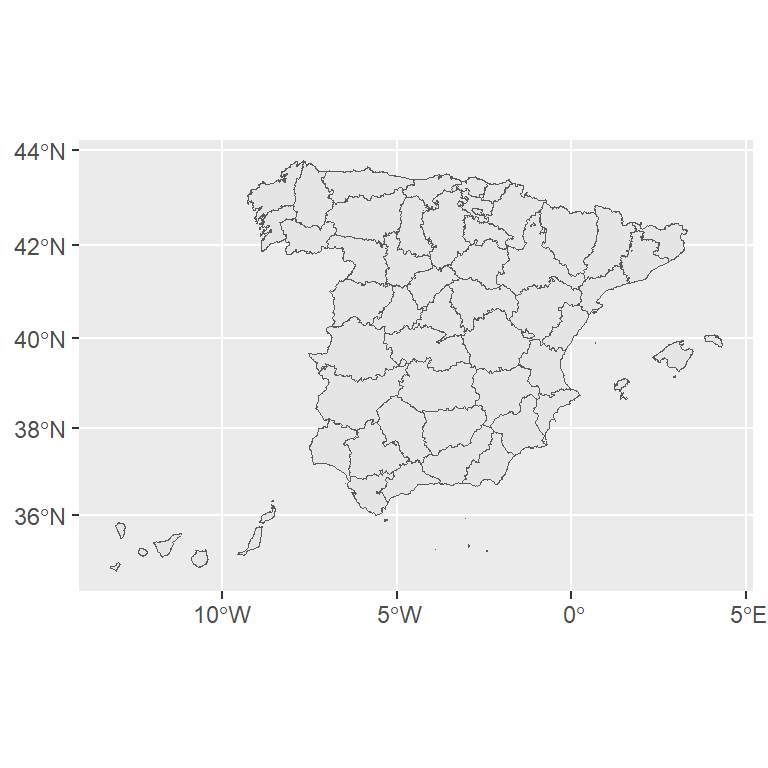# Cartograms in ggplot2

#### Author

Sebastian Jeworutzki

A cartogram is a type of map where different geographic areas are modified based on a variable associated to each of those areas. While cartograms can be visually appealing, they require a previous knowledge of the geography represented, since sizes and limits of the geographies are altered.

We would use the data included on the `mapSpain` package, that provides map data on `sf` format and an example dataset `pobmun19`, that includes the population of Spain by municipalty as of 2019.

## Base map

In first place, we would need to get the spatial data that contains the geographical information to be used on the plot. On `mapSpain`, we can select the provinces, that are the second-level administrative division of the country, and visualize the object with `geom_sf`:

``````# install.packages("sf")
library(sf)
# install.packages("dplyr")
library(dplyr)
# install.packages("ggplot2")
library(ggplot2)
# install.packages("mapSpain")
library(mapSpain)
# install.packages("cartogram")
library(cartogram)

# Data
prov <- esp_get_prov() %>%
mutate(name = prov.shortname.en) %>%
select(name, cpro)

# Base map
ggplot(prov) +
geom_sf()``````## Projection

We are going to use the `cartogram` package, that is dedicated to this specific task. Since `cartogram` requires a projected `sf` object, we would project our map to the well-known Mercator projection (EPSG code: 3857).``````# Transform the shape
prov_3857 <- st_transform(prov, 3857)

ggplot(prov_3857) +
geom_sf()``````

## Join map and data

In order to create a cartogram we will need to join the statistical and the geographical data. For that purpose, as the `sf` objects behave as data frames, we can use the `left_join` function from `dplyr`. Since the `pobmun19` dataset provides data at municipalty level, we need to aggregate this to the province level first:

``````# Aggregate
pop_provinces <- mapSpain::pobmun19 %>%
group_by(cpro) %>%
summarise(n_pop = sum(pob19))

prov_3857_data <- prov_3857 %>%
left_join(pop_provinces, by = c("cpro"))``````

After merging the data sets we would have an object with the data from `mapSpain::pobmun19` and a `geometry` column, that includes the geographical data coordinates.